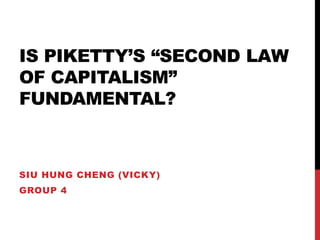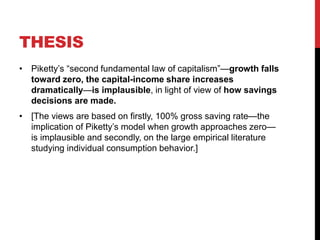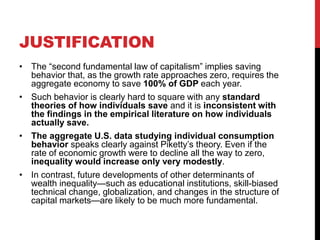Successfully reported this slideshow.

# Is Thomas Piketty’s ‘Second Law of Capitalism’ fundamental?×

# Is Thomas Piketty’s ‘Second Law of Capitalism’ fundamental?

A presentation on the Economic paper 'Is Piketty’s ‘Second Law of Capitalism’ fundamental?' by Krusell and Smith 2014.

A presentation on the Economic paper 'Is Piketty’s ‘Second Law of Capitalism’ fundamental?' by Krusell and Smith 2014.

### Is Thomas Piketty’s ‘Second Law of Capitalism’ fundamental?

1. 1. IS PIKETTY’S “SECOND LAW OF CAPITALISM” FUNDAMENTAL? SIU HUNG CHENG (VICKY) GROUP 4
2. 2. THESIS • Piketty’s “second fundamental law of capitalism”—growth falls toward zero, the capital-income share increases dramatically—is implausible, in light of view of how savings decisions are made. • [The views are based on firstly, 100% gross saving rate—the implication of Piketty’s model when growth approaches zero— is implausible and secondly, on the large empirical literature studying individual consumption behavior.]
3. 3. JUSTIFICATION • The “second fundamental law of capitalism” implies saving behavior that, as the growth rate approaches zero, requires the aggregate economy to save 100% of GDP each year. • Such behavior is clearly hard to square with any standard theories of how individuals save and it is inconsistent with the findings in the empirical literature on how individuals actually save. • The aggregate U.S. data studying individual consumption behavior speaks clearly against Piketty’s theory. Even if the rate of economic growth were to decline all the way to zero, inequality would increase only very modestly. • In contrast, future developments of other determinants of wealth inequality—such as educational institutions, skill-biased technical change, globalization, and changes in the structure of capital markets—are likely to be much more fundamental.
4. 4. SUPPORT: DERIVING THE NET SAVING RATE FROM OPTIMIZATION • Discuss whether Piketty’s assumed saving behavior can be rationalized based on intertemporal optimization adopting the most commonly used setting is one with infinitely-lived dynasties. Three ways: • First look at the simplest possible optimal-saving problem: one in partial equilibrium where the interest rate as well as the wage rate are constant. Then look at a general-equilibrium economy where the production technology is that assumed by Piketty. • In both cases, optimal behavior entails setting the net saving rate s ̃, as defined by Piketty, equal to zero when g = 0. • Introduce growth into a general equilibrium model of optimal saving with a standard production technology and report quantitatively how the gross and net savings rates vary with g on a balanced growth path. There we also briefly compare the various models of saving that we consider in this paper with observed U.S. data on gross and net savings rates.
5. 5. PIKETTY’S SECOND LAW OF CAPITALISM • Defining net saving , these assumptions deliver • Thus for all values of g, provided s ̃ > 0, capital is always increasing, because net output, F ̃, is positive. This is true even if g is 0, or less than 0! Piketty’s assumptions, in effect, amount to more “aggressive” saving behavior than in the textbook model. • With the same kind of transformation as in the standard model, we obtain • provided g > 0 we now obtain a steady-state level of kˆ such that • This law states that in the long run the wealth-to-income ratio equals s/g, and the wealth-to-income ratios are poised to increase dramatically as economies’ growth rates fall during the twenty-first century.
6. 6. DERIVING THE NET SAVING RATE FROM THE TEXTBOOK MODEL • Assuming that the textbook model describes saving behavior correctly, we can compute the net saving rate as defined by Piketty. It becomes • On a balanced growth path, and using the value of the ratio of capital to gross output from the textbook model, we thus obtain
7. 7. DERIVING THE NET SAVING RATE FROM THE TEXTBOOK MODEL • Using the ‘Second Law of Capitalism holding s ̃ constant would lead one to believe that a halving of the growth rate would lead to a doubling of k/y ̃ rather than less than a 30% increase. • This is no trivial matter because a key pillar of Piketty’s argument is that rates of economic growth are slowing to zero, leading therefore eventually to dramatic increases in the capital-to-net-income ratio, undermining the stability of capitalism.
8. 8. 1. A SINGLE CONSUMER • The consumer’s budget constraint: where w is a constant wage, 1 + r is the gross return from capital, and δ, as above, is the depreciation rate • Identify the consumer’s net, or “disposable”, income • We can identify net saving as a fraction of disposable income, s ̃, as 0, just as we just showed the textbook model would imply. Thus, we conclude that this model robustly predicts s ̃ = 0, along with a bounded value of k/y ̃. • The permanent-income model suggests that the “Piketty way” is consistent only if the relevant saving rate is zero, but this is precisely the rate that makes Piketty’s main argument—that the ratio of capital to net income explodes at g = 0—break down.
9. 9. 2. GENERAL EQUILIBRIUM WITH PIKETTY’S PRODUCTION FUNCTION • Piketty’s assumption is that F ̃ is positive so let us use the production function , which satisfies his assumption. This is a model with no depreciation and allows unbounded growth: • A problem that is concave and has a unique solution characterized by the usual, modern-macro Euler equation: • The Euler equation admits a steady state k defined uniquely by the condition
10. 10. 2. GENERAL EQUILIBRIUM WITH PIKETTY’S PRODUCTION FUNCTION • Even with production function used by Piketty—one that admits unbounded growth without technical change— standard assumptions on behavior delivers a steady state, quite in contrast with Piketty’s assumption on saving. • His assumption on saving is that s ̃ > 0, which the above analysis shows must not hold, but rather s ̃ = 0 is optimal in the long run. This, again, must obviously hold since net saving is kt+1 − kt and this is zero whenever the economy reaches a steady state.
11. 11. 3. POSTWAR U.S. DATA • Data used is postwar U.S. annual time series for output and net and gross saving rates since 1950 • Recall that in Piketty’s model, the net saving rate is constant over time and independent of the growth rate g, implying that as growth increases, the implied gross saving rate declines • In contrast, the textbook version of the Solow model has the gross saving rate constant, with the implied net saving rate responding positively to the growth rate
12. 12. 3A. RELATIONSHIP BETWEEN SAVING RATES AND GROWTH in the usual optimizing growth model with a standard production function, straightforward calculations show that both saving rates (net and gross) are increasing in g.
13. 13. 3B. OBSERVED SAVING RATES OVER TIME
14. 14. 3B. OBSERVED SAVING RATES OVER TIME • We observe that at least in percentage terms, the fluctuations in s ̃ are larger than those in s. In this sense, if one were to choose between making one of them constant over time, it would make more sense to assume s constant: the textbook version of the Solow model. • We also see that s ̃ has fallen gradually toward zero; it was below zero during the recent recession and over the last 5 or so years is well approximated by zero. Thus, that s ̃ will remain constant and positive in the twenty-first century does not appear like a good assumption at all.
15. 15. 3C. HOW SAVING RATES APPEAR TO VARY WITH GROWTH RATES
16. 16. C. HOW SAVING RATES APPEAR TO VARY WITH GROWTH RATES • There is a strong positive relationship between saving rates and growth rates. The data is therefore consistent with the optimizing theory in this sense. • We see also that the intercept for s ̃, at g = 0, is negative, if anything, not positive. Thus, projecting into the future based on Piketty’s second fundamental law, with g going to zero, appears unwise.
17. 17. COMMENTS ON PIKETTY’S POINT OF VIEW • Piketty’s assumptions on saving are non-standard, relative to the applied economics literature, a comparison with the standard model ought to be a main concern in his works, where he does appear to claim that his model allows an accurate account of the historical data. • Piketty and Zucman (2014) studies capital accumulation in a cross-section of countries from the perspective of his formulation of aggregate saving but does not address the central question of how net saving rates vary with growth rates. Tables 3 and 4 in this paper report net saving rates and growth rates in a small cross-section of developed countries for the period 1970–2010; here there seems to be no systematic relationship between net saving rates and growth rates.
18. 18. COMMENTS ON PIKETTY’S POINT OF VIEW • Piketty himself notes in his work, countries differ in all sorts of ways (attitudes towards saving, institutional structures for savings, etc.) that one would want to control for. • What would be required instead, expanding on our analysis of U.S. data, is a panel study of a cross-section countries with country-specific fixed effects using, if possible, data going much further back in time. • Such an analysis is beyond the scope of this paper, but we guess that such data will imply that low-growth episodes are associated with low, not high, gross saving rates
19. 19. CONCLUSION • The optimal-saving model—that is, the model used in the applied microeconomics literature and by Cass and Koopmans in a growth context—seems to fit the U.S. postwar data the best. Piketty’s model, on the other hand, does not appear consistent with this data. • Using these models as a basis for prediction, we robustly find very modest effects of a declining growth rate on the capital-output ratio and on inequality.
20. 20. CONCLUSION • It was not until researchers started to take “microfoundations” seriously—specifying explicitly the decision problem faced by savers and identifying the state variables such as capital, upon which their decisions depend—that some of the confusions about how to specify saving rules began to clear up. • The Solow growth model has universally assumed a constant gross saving rate, and we have argued that in an environment without growth in either population or technology this is the only assumption that makes sense.